# 341

## Summary

### Factorization

The factorization is as follows, with factors 11 and 31: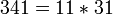### Properties and families

Property or family Parameter values First few members of the family Proof of satisfaction/membership/containment
Poulet number (also called Sarrus number), i.e., Fermat pseudoprime to base 2 smallest Poulet number 341, 561, 645, 1105, 1387, 1729, 1905, 2047, [SHOW MORE] View list on OEIS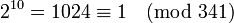, so.

## Arithmetic functions

Function Value Explanation
Euler totient function 300 The Euler totient function is.
universal exponent 30 The universal exponent is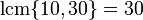.
divisor count function 4where the first 1s in both factors are the multiplicities of the prime divisors.
divisor sum function 384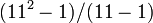times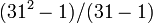equals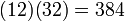.
Mobius function 1 The number is square-free and has an even number of prime divisors (2 prime divisors).

## Structure of integers mod 341

### Abstract structure

The discussion here about the multiplicative group of integers modulo n relies on some background facts from group theory.
FACTS ABOUT MULTIPLICATIVE GROUPS: Classification of natural numbers for which the multiplicative group is cyclic | Characterization of multiplicative group of integers modulo n
GENERAL FACTS ABOUT GROUPS: order of direct product is product of orders, exponent of direct product is lcm of exponents

By the Chinese remainder theorem, we have the ring decomposition:For the multiplicative group, this gives: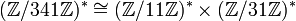Both groups on the right side are cyclic with orders 10 and 30 respectively, so the whole group is: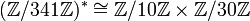This group has order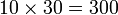(the order of the multiplicative group is termed the Euler totient function and denoted, so this is saying that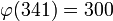).

The exponents of the factor groups are 10 and 30 respectively, hence the exponent of the whole group is the lcm of these, which is 30. The exponent of the multiplicative group is termed the universal exponent and denoted, so this is saying that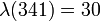).

## Primality testing

### Summary

Primality test Relies on ... Total number of candidate bases Number of bases wih gcd more than 1 Number of witnesses that are relatively prime Number of liars that are relatively prime Total number of witnesses Probability of picking witness Probability of picking liar Conditional probability of picking witness for relatively prime Conditional probability of picking liar for relatively prime
Fermat primality test Fermat's little theorem 340 40 200 100 240 240/340 = 12/17 100/340 = 5/17 2/3 1/3
Solovay-Strassen primality test Euler's criterion 340 40  ?  ?  ?  ?  ?  ?  ?
Miller-Rabin primality test 340 40  ?  ?  ?  ?  ?  ?  ?

### Fermat test

341 is a Fermat pseudoprime to baseif the order ofmod 11 divides 340 and the order ofmod 31 divides 340. The first condition always holds, because 11 - 1 = 10 divides 340. The second condition is equivalent to the order dividing 10, which holds for 1/3 of the bases relatively prime to 31. Overall, 341 is a Fermat pseudoprime for 1/3 of the 300 possible bases relatively prime to it (i.e., a total of 100 possible bases). This means that if we use the Fermat test for a base picked at random, there is a 2/3 chance that we will be able to use it to conclude that 341 is not prime.

2 is one of the bases for which 341 is a Fermat pseudoprime, because. Thus, 341 is a Poulet number or Sarrus number.

### Euler test

Fill this in later

### Strong Fermat test

Fill this in later